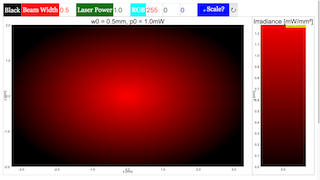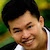Intro Page

Laser Beam Profile

Developed by E. Behringer

This set of exercises guides the student to model the results of an experiment to determine the profile of a laser beam using a knife-edge technique. It requires the development of the model of the knife-edge profile, and fitting of the model profile to experimental data. Here, the computational tasks are handled by built-in functions of the computational tool being used to complete these exercises.

 Subject Area Waves & Optics Beyond the First Year Python Students who complete this set of exercises will be able to express an equation predicting the profile of a laser oscillating in the TEM00 mode in terms of dimensionless (“scaled”) variables suitable for coding (Exercise 1); produce both line plots and contour plots of the (scaled) irradiance of the beam versus (scaled) position(s) (Exercises 1 and 2); develop a model of, and plot, the knife-edge profile of the laser beam (Exercise 3); and fit the model, i.e., of the irradiance versus knife-edge position, to experimental data (Exercise 4) 120 min
Beam_Profile_Exercise_2.py

#

# Beam_Profile_Exercise_2.py

#

# This file will generate a filled contour plot of

# the irradiance I(x,y) of the TEM00 Gaussian Mode of a laser

# versus x and y.

#

# This file will also generate a filled contour plot of

# the scaled irradiance I(x,y)/I_max of the TEM00 Gaussian Mode of a laser

# versus scaled x and scaled y.

#

# Written by:

#

# Ernest R. Behringer

# Department of Physics and Astronomy

# Eastern Michigan University

# Ypsilanti, MI 48197

# (734) 487-8799

# This email address is being protected from spambots. You need JavaScript enabled to view it.

#

# with contributions from graduate student

#

# Najwa Sulaiman

# Department of Physics and Astronomy

# Eastern Michigan University

# Ypsilanti, MI 48197

# This email address is being protected from spambots. You need JavaScript enabled to view it.

#

# 20160529 ERB

#

from pylab import xlim,xlabel,ylim,ylabel,show,contourf,colorbar,figure,title

from matplotlib.colors import LinearSegmentedColormap

from numpy import linspace,meshgrid,exp,sqrt,pi,amax

# Define the colormaps for these contour plots.

# For a HeNe laser beam profile, we might want red to be bright

# and black to be dark. This is the color map labeled

# black_red1.

#

# If instead we want white to be dark,

# then we use the color map labeled white_red1.

#

# The first digit in each 3-tuple is the scaled intensity

# (0 for lowest value, 1 for highest value).

# The second two digits are identical and represent the

# R-, G-, or B-value at that scaled intensity. Here,the

# R-value increases linearly with intensity while the

# G- and B-values are always zero.

cdict1 = {'red': ((0.0, 0.0, 0.0),

(1.0, 1.0, 1.0)),

'green': ((0.0, 0.0, 0.0),

(1.0, 0.0, 0.0)),

'blue': ((0.0, 0.0, 0.0),

(1.0, 0.0, 0.0))}

black_red1 = LinearSegmentedColormap('Black_Red1',cdict1)

#

cdict2 = {'red': ((0.0, 1.0, 1.0),

(1.0, 1.0, 1.0)),

'green': ((0.0, 1.0, 1.0),

(1.0, 0.0, 0.0)),

'blue': ((0.0, 1.0, 1.0),

(1.0, 0.0, 0.0))}

white_red1 = LinearSegmentedColormap('White_Red1',cdict2)

#x and y array values to be used later

max_x = 2.0 # maximum value of x position [mm]

min_x = -2.0 # minimum value of x position [mm]

max_y = max_x # maximum value of y position [mm]

min_y = min_x # minimum value of y position [mm]

Npts = 81

x = linspace(min_x,max_x,Npts) #x data point array

y = linspace(min_y,max_y,Npts) #y data point array

# create mesh of points on which irradiance is evaluated

X, Y = meshgrid(x,y)

# Now scale the x and y values by the beam width w0

w0 = 0.5 # beam width w0 [mm]

scaled_x = x/w0

scaled_y = y/w0

#creating grid table for function to be set on

Xsc, Ysc = meshgrid(scaled_x,scaled_y)

# other inputs

P_0 = 1.00 # laser power [mW]

I_0 = 2.00*P_0/(pi*w0*w0) # maximum irradiance [mW/mm2]

I = I_0 * exp(-2.0*(X*X + Y*Y))

Imax = amax(I)

Isc = I/Imax

# Generate the contour plot of irradiance

# versus x and y with black as dark and red as bright

figure()

# Use the colormap black_red1

contourf(X,Y,I,100,cmap=black_red1)

# Define the limits of the horizontal axis

xlim(min_x,max_x)

# Label the horizontal axis, with units

xlabel("$$x$$ [mm]", size = 16)

# Define the limits of the vertical axis

ylim(min_y,max_y)

# Label the vertical axis, with units

ylabel("$$y$$ [mm]", size = 16)

# Plot title

title('$$w_0 =$$%s mm, $$P_0 =$$%s mW'%(w0,P_0))

# Show the colorbar

colorbar(label='Irradiance [mW/mm$$^2$$]')

show()

# Generate the contour plot of scaled irradiance

# versus scaled x and scaled y with black as dark and red as bright

figure()

# Use the colormap black_red1

contourf(Xsc,Ysc,Isc,100,cmap=black_red1)

# Define the limits of the horizontal axis

xlim(min_x,max_x)

# Label the horizontal axis, with units

xlabel("$$\\tilde{x}\equiv x/w_0$$", size = 16)

# Define the limits of the vertical axis

ylim(min_y,max_y)

# Label the vertical axis, with units

ylabel("$$\\tilde{y}\equiv y/w_0$$", size = 16)

# Plot title

title('$$w_0 =$$%s mm, $$P_0 =$$%s mW'%(w0,P_0))

# Show the colorbar

show()

Exercise 2

### EXERCISE 2: THE TEM00MODE: A CONTOUR PLOT

(a) A filled contour plot is a plot that indicates value by color as a function of two variables. Make a plot of the irradiance versus the variables  and  for , with  mm and  mW.

(b) Using the same values for  and , make a filled contour plot of the scaled irradiance  versus the scaled variables  and  for .

### Translations

Code Language Translator Run### Software Requirements

SoftwareRequirements

 Android iOS Windows MacOS with best with Chrome Chrome Chrome Chrome support full-screen? Yes. Chrome/Opera No. Firefox/ Samsung Internet Not yet Yes Yes cannot work on some mobile browser that don't understand JavaScript such as..... cannot work on Internet Explorer 9 and below

### CreditsFremont Teng; Loo Kang Wee

[text]

[text]

Research

[text]

[text]

[text]

### end faq